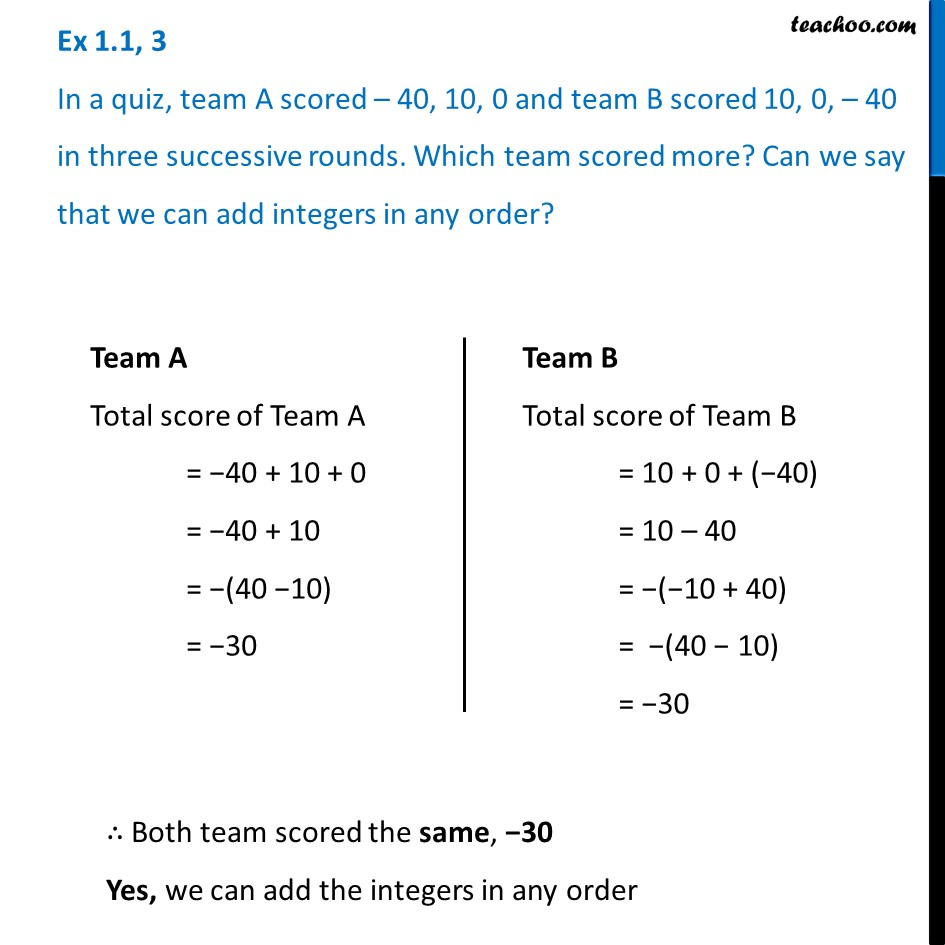Chapter 1 Class 7 Integers
Concept wiseLearn in your speed, with individual attention - Teachoo Maths 1-on-1 Class

### Transcript

Ex 1.1, 3 In a quiz, team A scored – 40, 10, 0 and team B scored 10, 0, – 40 in three successive rounds. Which team scored more? Can we say that we can add integers in any order?Team A Total score of Team A = −40 + 10 + 0 = −40 + 10 = −(40 −10) = −30 Team B Total score of Team B = 10 + 0 + (−40) = 10 – 40 = −(−10 + 40) = −(40 − 10) = −30 ∴ Both team scored the same, −30 Yes, we can add the integers in any order